Study Guide

# Dimensions and Extensions

## Dimensions and Extensions

So far everything we've been doing has been flat, boring, two-dimensional. It's time to add some depth to things (and to show how hard it is to draw depth on a sheet of paper or a computer screen).

As you've hopefully noticed at some point in your life, the world is more than a single sheet of paper. In fact, you can have several different sheets of paper, oriented in several different ways. But this is about geometry, not the state of your backpack.

• ### Planes

A plane is like an infinitely big sheet of paper that continues forever in all directions. It's sort of like a single slice of the three-dimensional world in the same way that a line is like a single slice of a sheet of paper.

If you want to get philosophical about it, we say that the universe we live in is three-dimensional, each slice of that (each plane) is two-dimensional, each slice of those slices (each line) is one-dimensional, and sometimes just to be ridiculous we say points are zero-dimensional.

Planes go on forever like lines do. We can't draw planes that extend forever and ever, so we use borders to represent them. Unlike lines, we name planes with super-fancy cursive capital letters. You may want to brush up on your calligraphy skills.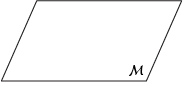Sometimes it's hard to tell from a picture whether a plane contains a given line or a point, so we try to make the drawings very obvious.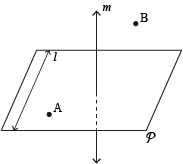Here, plane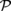contains line l and point A, but not line m or point B.

Any three points can be contained in a single plane. To visualize this, imagine a triangle connecting the three points, and simply extend the boundary of the triangle to get a plane. However, if you have more than three points, they might not live on a single plane. A square pyramid is an excellent example of this.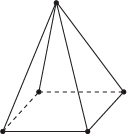The only plane containing four points is the bottom of the pyramid, and that plane will never contain the vertex of the pyramid. When a bunch of points lie on the same line, we call them collinear, so it only makes sense that when a bunch of points or lines lie on the same plane, we call them coplanar.

### Sample Problem

Suppose we have a bunch of collinear points. Are they coplanar?

It might seem like we don't have enough information to answer this question, but it turns out that we do. No matter how many points we had, we know for a fact that we can capture collinear points by a single line.

That means if we choose any plane containing that line (and we have infinitely many to choose from just by rotating the plane around the line), that plane must contain all the points. That means the collinear points are also coplanar.

Mathematicians sometimes abbreviate this phenomenon by saying something like "collinearity implies coplanarity" or "collinearity is stronger than coplanarity." To be fair, it has been working out a lot recently.

• ### Multiple Planes

The relationships between lines and planes are a bit complicated. As we mentioned, any single line can be contained in a plane. Sometimes, though, you have two lines that cannot be contained in a single plane.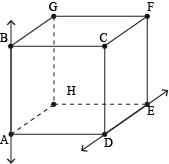AB and DE cannot be contained in a single plane. In this situation, we say the lines are skew. Skew lines are kind of weird—they don't intersect each other, but they're not quite parallel either. If parallel lines were friends and intersecting lines were enemies, skew lines would be people who have never met and don't care about each other one bit.

What about planes interacting with other planes? In three-dimensional space, planes are either parallel or intersecting (in higher dimensional spaces you can have skew planes, but that's too trippy to think about). Parallel planes never meet, looking kind of like this: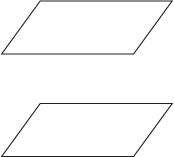Intersecting planes intersect each other. Shocker. They look a little something like this: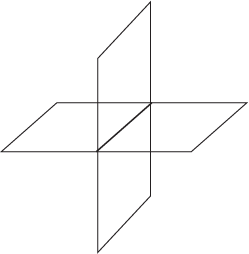Well, as we can see from the picture, the planes intersect in several points. In fact, they intersect in a whole line! If you imagine two intersecting planes as the monitor and keyboard of a laptop, their intersection is the line containing those flimsy joints that you're always paranoid airport security will break when inspecting your computer.Three planes can intersect at a point, but if we move beyond 3D geometry, they'll do all sorts of funny things. Fortunately, we won't go past 3D geometry. We just thought we should warn you in case you ever find yourself in an alternate universe or the seventh dimension thinking, "I wonder if planes here work the same way."

• ### Cartesian Coordinates

Remember back when we were musing philosophically about dimensions and slices and all that jazz? We weren't kidding about the philosophy. Much of that stuff was developed by René Descartes (famous for the oh-so-overquoted, "I think, therefore I am"). And he wasn't even Greek.

Descartes realized that coordinates can specify points, and geometrical relationships between the points can be interpreted as numerical relationships between their coordinates. That means we can have two coordinates and find the distance between 'em.

Let's start with a simple example: say we have a horizontal line l containing a point O.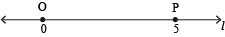We can give O the coordinate 0 (we sometimes call this starting point the origin). If point P is 5 units to the right of O, we can give P the coordinate 5. In general, we can use positive numbers for right or up, if the line is vertical, and negative numbers for left or down. Then we just write down how far the point is from O. If the line is diagonal, pick positive to be the direction that tends towards the right.

### Sample Problem

What is the coordinate for point X in the picture?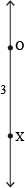Since X is 3 units away from O and is down from it, we should give X the coordinate -3.

Putting coordinates on lines really just makes them look like the familiar number line from algebra.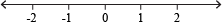Finding the distance between two points on the line is easy: it's the bigger coordinate minus the smaller coordinate. Distance should always be positive, so we tend to stick the answer in an absolute value sign just in case we subtracted the wrong way.

### Sample Problem

Suppose X has coordinate 4, and Y has coordinate -1. What is the length of the segment XY?

Let's start by drawing a picture: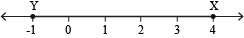Since the length of XY is just the distance from X to Y, we can compute it from the coordinates as

XY = |4 – (-1)| = |5| = 5.

What if we're trying to pinpoint a location on a piece of paper using coordinates? We can pick, say, the upper left corner as the origin, calling it O. But which point should get the coordinate 1? There are too many points of distance 1 from the origin to pick from.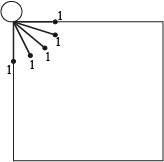We can't even get around this by using +1 and -1, since there are more than two options. However, we can pinpoint exactly one location by using two coordinates. For example, we can say point P is 0.8 units to the right and 0.6 units down from O.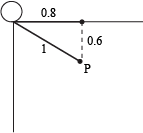This specifies P exactly, and we can say (0.8, -0,6) are the coordinates of P.

• ### Distance and Midpoint Formulas

Slapping coordinates on a line makes any line look like the number line. Just the same, this system of coordinates can make any plane look like the x-y plane from algebra.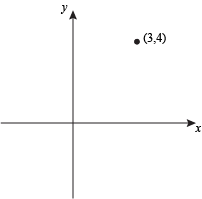Since lines only require one coordinate, they're one-dimensional. Planes require two, so they're two-dimensional. If we kick it up one more notch, we can put points in space (three-dimensional space, not the Final Frontier).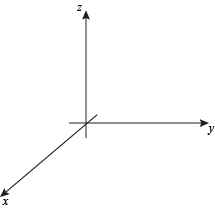Finally, if we already know the exact point we're talking about, there's no need to add any coordinates to specify anything. That's why points are called zero-dimensional.

Going back to two dimensions, we can use coordinates to find the distance between points using the distance formula: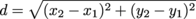### Sample Problem

If M = (3, 4) and N = (5, -2), find the length of the segment MN.

As always, it's a good idea to sketch the problem first.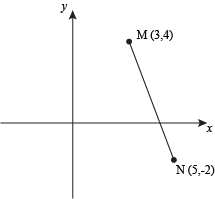Since the length of MN is just the distance between the endpoints, we can use the distance formula.Plugging this into a calculator, we get approximately 6.32 units, which looks about right on the sketch.

You might be wondering why computing one-dimensional distance looks so different from the more complicated 2D distance formula. Well, if we follow the pattern with the square root and everything using only one coordinate, we get: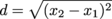d = |x2x1|

The square root cancels out the exponent, but we have to put absolute values since square roots, just like distances, are always positive. Basically, they're the same concepts extended to multiple dimensions.

The distance formula is really just an adaptation of the infamous Pythagorean Theorem: a2 + b2 = c2. We'll talk about this more when we get into right triangles. We just said it because Greek influence extends even to Descartes's work. Geometry really is all about the Greeks, isn't it?

Coordinates also make it easy to find the midpoint of a segment. Finding the number in the middle of two others means finding their average, in exactly the same way that the average of a and b is (a + b) ÷ 2. Average each of the coordinates of the endpoints, and you've got a midpoint. Here's a formula, if you prefer to remember those: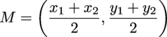### Sample Problem

Line segment PQ has length 10 and has M as a midpoint. If P has coordinates (2, 4) and M has coordinates (5, 8), what are the coordinates of point Q?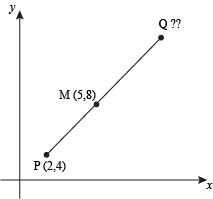It's tempting to plug (2, 4) and (5, 8) into the midpoint formula, but what would that give us? We'd just get the midpoint of P and M, which doesn't answer the question at all.

Instead, we're given a midpoint and need to solve for an endpoint. Let's just call the coordinates of Q (x, y) for now. We can still use the midpoint formula, but not in the same way we'd want to.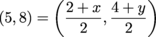This looks like a single equation, but it's actually two equations disguised as one. We know that the first coordinates have to be equal, and also that the second coordinates are equal. In other words, we have these two equations: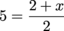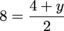Solving for x and y (or just guessing and checking) yields (x, y) = (8, 12), and we're done.

Hold up. We never used the length of PQ. Does that mean our answer is wrong? Not necessarily. Many times in geometry, we're given extra information to try to throw us off. Sometimes it's completely irrelevant ("If 1 + x = 83 and an orangutan's arms can extend up to 7 feet, find the value of x"). Sometimes it's actually pertinent to the question, but not needed to solve the problem. When that happens, we can use this extra information to check our answer.

In this case, we can check and see if the distance between P and Q is 10. If it is, then we have more proof (not proofs again!) that our answer is right. If we're wrong, we may want to go back and check our work.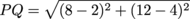PQ = 10

We're right, so there's no need to worry.